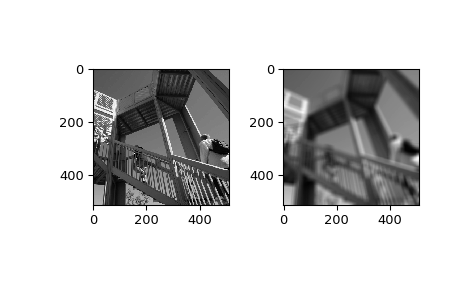# scipy.ndimage.uniform_filter¶

scipy.ndimage.uniform_filter(input, size=3, output=None, mode='reflect', cval=0.0, origin=0)[source]

Multi-dimensional uniform filter.

Parameters
inputarray_like

The input array.

sizeint or sequence of ints, optional

The sizes of the uniform filter are given for each axis as a sequence, or as a single number, in which case the size is equal for all axes.

outputarray or dtype, optional

The array in which to place the output, or the dtype of the returned array. By default an array of the same dtype as input will be created.

modestr or sequence, optional

The mode parameter determines how the input array is extended when the filter overlaps a border. By passing a sequence of modes with length equal to the number of dimensions of the input array, different modes can be specified along each axis. Default value is ‘reflect’. The valid values and their behavior is as follows:

‘reflect’ (d c b a | a b c d | d c b a)

The input is extended by reflecting about the edge of the last pixel.

‘constant’ (k k k k | a b c d | k k k k)

The input is extended by filling all values beyond the edge with the same constant value, defined by the cval parameter.

‘nearest’ (a a a a | a b c d | d d d d)

The input is extended by replicating the last pixel.

‘mirror’ (d c b | a b c d | c b a)

The input is extended by reflecting about the center of the last pixel.

‘wrap’ (a b c d | a b c d | a b c d)

The input is extended by wrapping around to the opposite edge.

cvalscalar, optional

Value to fill past edges of input if mode is ‘constant’. Default is 0.0.

originint or sequence, optional

Controls the placement of the filter on the input array’s pixels. A value of 0 (the default) centers the filter over the pixel, with positive values shifting the filter to the left, and negative ones to the right. By passing a sequence of origins with length equal to the number of dimensions of the input array, different shifts can be specified along each axis.

Returns
uniform_filterndarray

Filtered array. Has the same shape as input.

Notes

The multi-dimensional filter is implemented as a sequence of one-dimensional uniform filters. The intermediate arrays are stored in the same data type as the output. Therefore, for output types with a limited precision, the results may be imprecise because intermediate results may be stored with insufficient precision.

Examples

>>> from scipy import ndimage, misc
>>> import matplotlib.pyplot as plt
>>> fig = plt.figure()
>>> plt.gray()  # show the filtered result in grayscale
>>> ax1 = fig.add_subplot(121)  # left side
>>> ax2 = fig.add_subplot(122)  # right side
>>> ascent = misc.ascent()
>>> result = ndimage.uniform_filter(ascent, size=20)
>>> ax1.imshow(ascent)
>>> ax2.imshow(result)
>>> plt.show()#### Previous topic

scipy.ndimage.sobel

#### Next topic

scipy.ndimage.uniform_filter1d